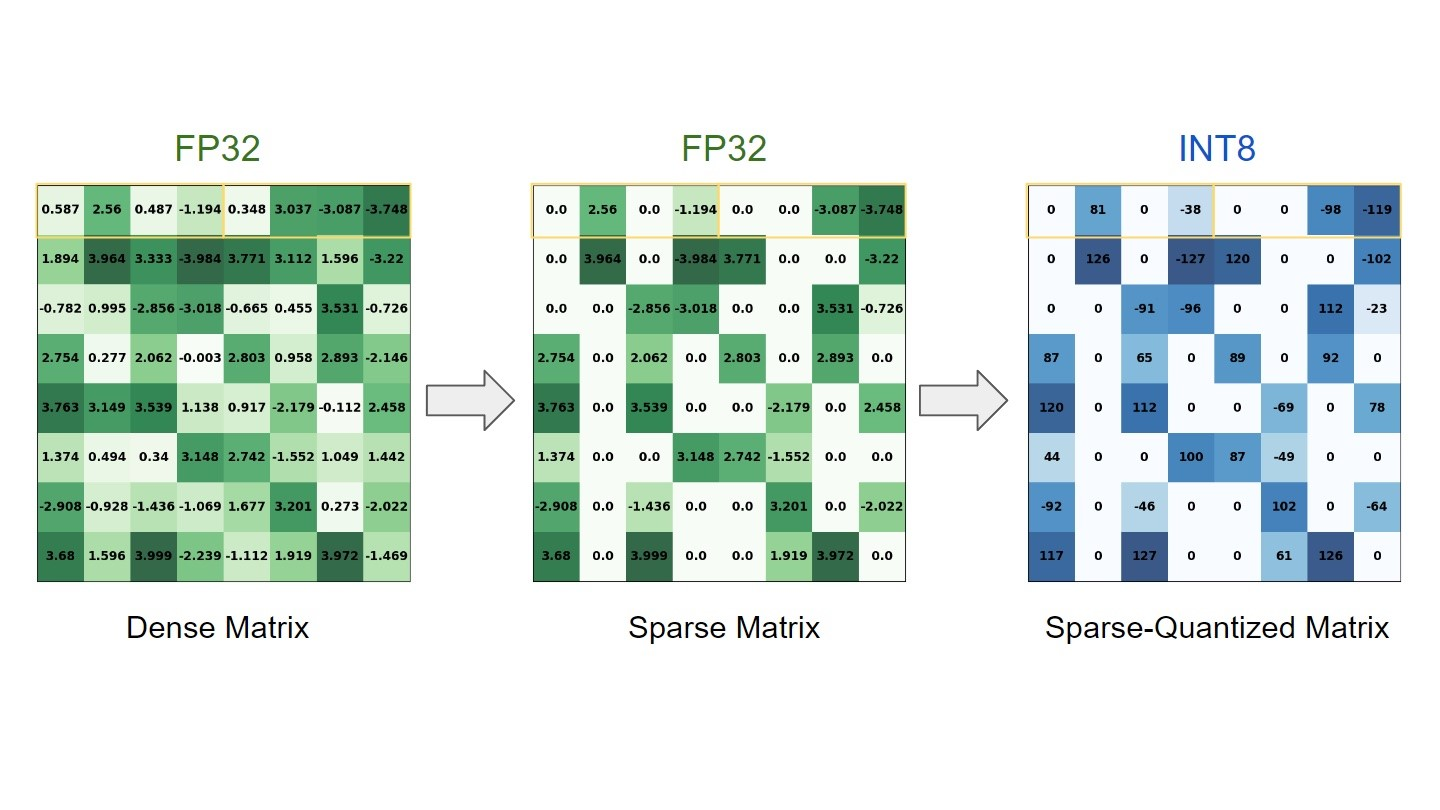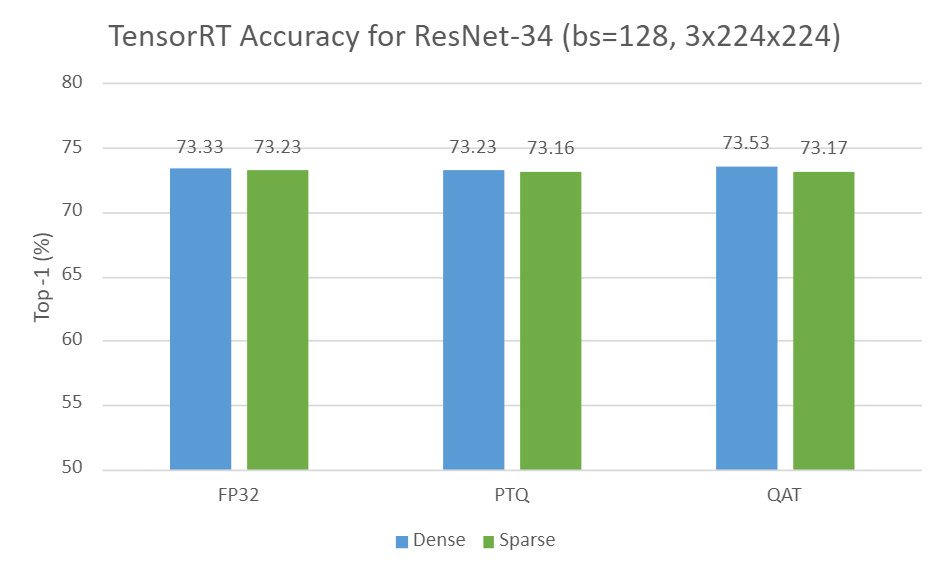## INT8 中的稀疏性：加速的训练工作流程和NVIDIA TensorRT 最佳实践

news/2023/9/26 7:37:21

# INT8 中的稀疏性：加速的训练工作流程和NVIDIA TensorRT 最佳实践### 文章目录

• INT8 中的稀疏性：加速的训练工作流程和NVIDIA TensorRT 最佳实践
• 结构稀疏
• 量化
• 在 TensorRT 中部署稀疏量化模型的工作流程
• 案例研究：ResNet-34
• 要求
• 第 1 步：从密集模型中进行稀疏化和微调
• 第 2 步：量化 PyTorch 模型
• PTQ 通过 TensorRT 校准
• QAT 通过 pytorch-quantization 工具包
• 第三步：部署TensorRT引擎
• 结果

NVIDIA TensorRT 已经提供量化支持一段时间（从 2.1 版本开始），并且最近在 NVIDIA Ampere 架构 Tensor Cores 中内置了对稀疏性的支持，并在 TensorRT 8.0 中引入。

## 结构稀疏

NVIDIA 稀疏张量核心使用 2:4 模式，这意味着四个值的每个连续块中的两个必须为零。 换句话说，我们遵循 50% 的细粒度结构化稀疏度配方，由于直接在 Tensor Core 上提供可用支持，因此不会对零值进行任何计算。 这导致在相同的时间内计算更多的工作量。 在这篇文章中，我们将此过程称为修剪。

## 量化

• 训练后量化 (PTQ)：使用隐式量化工作流程。 在隐式量化网络中，每个量化张量都有一个关联的标度，用于通过校准对值进行隐式量化和反量化。 然后 TensorRT 检查该层运行速度更快的精度并相应地执行它。
• 量化感知训练 (QAT)：使用显式量化工作流程。 显式量化网络利用量化和反量化 (Q/DQ) 节点来明确指示必须量化哪些层。 这意味着您可以更好地控制在 INT8 中运行哪些层。 有关详细信息，请参阅 Q/DQ 层布局建议。

## 在 TensorRT 中部署稀疏量化模型的工作流程

• 在 PyTorch 中对预训练的密集模型进行稀疏化和微调。
• 通过 PTQ 或 QAT 工作流程量化稀疏化模型。
• 在 TensorRT 中部署获得的稀疏 INT8 引擎。
下图显示了所有三个步骤。 步骤 2 中的一个区别是 Q/DQ 节点存在于通过 QAT 生成的 ONNX 图中，但在通过 PTQ 生成的 ONNX 图中不存在。 有关详细信息，请参阅使用 INT8。

• 在 PyTorch 中对预训练的密集模型进行稀疏化和微调。
• 在 PyTorch 中量化、校准和微调稀疏模型。
• 将 PyTorch 模型导出到 ONNX。
• 通过 ONNX 生成 TensorRT 引擎。
• 在 TensorRT 中部署获得的 Sparse INT8 引擎。

• 在 PyTorch 中对预训练的密集模型进行稀疏化和微调。
• 将 PyTorch 模型导出到 ONNX。
• 通过 TensorRT 构建器对稀疏化的 ONNX 模型进行校准和量化，生成 TensorRT 引擎。
• 在 TensorRT 中部署获得的稀疏 INT8 引擎。## 案例研究：ResNet-34

### 要求

• Python 3.8
• PyTorch 1.11 (also tested with 2.0.0)
• PyTorch vision
• apex sparsity toolkit
• pytorch-quantization toolkit
• TensorRT 8.6
• Polygraphy
• ONNX opset>=13
• NVIDIA Ampere architecture GPU for Tensor Core support

### 第 1 步：从密集模型中进行稀疏化和微调

``````import copy
from torchvision import models
from apex.contrib.sparsity import ASP# Load dense model
model_dense = models.__dict__["resnet34"](pretrained=True)# Initialize sparsity mode before starting sparse training
model_sparse = copy.deepcopy(model_dense)
ASP.prune_trained_model(model_sparse, optimizer)# Re-train model
for e in range(0, epoch):for i, (image, target) in enumerate(data_loader):image, target = image.to(device), target.to(device)output = model_sparse(image)loss = criterion(output, target)optimizer.zero_grad()loss.backward()optimizer.step()# Save model
torch.save(model_sparse.state_dict(), "sparse_finetuned.pth")
``````

### 第 2 步：量化 PyTorch 模型

#### PTQ 通过 TensorRT 校准

``````dummy_input = torch.randn(batch_size, 3, 224, 224, device="cuda")
torch.onnx.export(model_sparse, dummy_input, "sparse_finetuned.onnx", opset_version=13, do_constant_folding=True)``````

``````from infer_engine import infer
from polygraphy.backend.trt import Calibrator, CreateConfig, EngineFromNetwork, NetworkFromOnnxPath, TrtRunner, SaveEngine
from polygraphy.logger import G_LOGGER# Data loader argument to `Calibrator`
def calib_data(val_batches, input_name):for iteration, (images, labels) in enumerate(val_batches):yield {input_name: images.numpy()}# Set path to ONNX model
onnx_path = "sparse_finetuned.onnx"# Set calibrator
calibration_cache_path = onnx_path.replace(".onnx", "_calibration.cache")
)# Build engine from ONNX model by enabling INT8 and sparsity weights, and providing the calibrator
build_engine = EngineFromNetwork(NetworkFromOnnxPath(onnx_path),config=CreateConfig(int8=True,calibrator=calibrator,sparse_weights=True)
)# Trigger engine saving
engine_path = onnx_path.replace(".onnx", ".engine")
build_engine = SaveEngine(build_engine, path=engine_path)# Calibrate engine (activated by the runner)
with G_LOGGER.verbosity(G_LOGGER.VERBOSE), TrtRunner(build_engine) as runner:print("Calibrated engine!")# Infer PTQ engine and evaluate its accuracylog_file = engine_path.split("/")[-1].replace(".engine", "_accuracy.txt")infer(engine_path,data_loader_test,batch_size=args.batch_size,log_file=log_file)``````

#### QAT 通过 pytorch-quantization 工具包

``from apex.contrib.sparsity import ASPdef prune_trained_model_custom(model, optimizer, compute_sparse_masks=False):asp = ASP()asp.init_model_for_pruning(model, mask_calculator="m4n2_1d", verbosity=2, whitelist=[torch.nn.Linear, torch.nn.Conv2d], allow_recompute_mask=False)asp.init_optimizer_for_pruning(optimizer)if compute_sparse_masks:asp.compute_sparse_masks()``

``````
from pytorch_quantization import nn as quant_nnclass BasicBlock(nn.Module):def __init__(self, ..., quantize: bool = False) -> None:super().__init__()...if self._quantize:self.residual_quantizer = quant_nn.TensorQuantizer(quant_nn.QuantConv2d.default_quant_desc_input)def forward(self, x: Tensor) -> Tensor:identity = x...if self._quantize:out += self.residual_quantizer(identity)else:out += identityout = self.relu(out)return out
``````

``````# Add Q/DQ nodes to the dense model
from pytorch_quantization import quant_modules
quant_modules.initialize()
model_qat = models.__dict__["resnet34"](pretrained=True, quantize=True)# Initialize sparsity mode before starting Sparse-QAT fine-tuning
compute_amax(model_qat, method="entropy”)# Fine-tune model
for e in range(0, epoch):for i, (image, target) in enumerate(data_loader):image, target = image.to(device), target.to(device)output = model_qat(image)...# Save model
torch.save(model_qat.state_dict(), "quant_finetuned.pth")``````

``````from pytorch_quantization import nn as quant_nn
quant_nn.TensorQuantizer.use_fb_fake_quant = True
dummy_input = torch.randn(batch_size, 3, 224, 224, device="cuda")
torch.onnx.export(model_qat, dummy_input, "quant_finetuned.onnx", opset_version=13, do_constant_folding=True)``````

``````\$ trtexec --onnx=quant_finetuned.onnx --int8 --sparsity=enable --saveEngine=quant_finetuned.engine --skipInference
``````

### 第三步：部署TensorRT引擎

``````\$ trtexec --loadEngine=quant_finetuned.engine -v
``````

## 结果

• FP32 中的密集与稀疏
• INT8 中的密集 PTQ 与稀疏 PTQ
• INT8 中的密集 QAT 与稀疏 QAT### 图论试题2021

25 A&#xff1a;最大度是7&#xff0c;大于了顶点数6&#xff0c;故不是简单图的度序列。 C&#xff1a;树的度序列至少要有两个度为1的顶点 D&#xff1a;只要度数为奇数的个数有偶数个&#xff0c;就是度序列。 A&#xff1a;每棵树的中心由一个点或两个相邻点组成 B&…